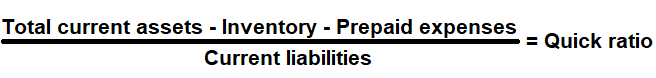# Quick Ratio Calculator

Key in the Current Assets, Inventory and Current Liabilities Values from your Balance Sheet to the respective fields given below and then click Calculate to get the desired result.

# Quick Ratio Calculator - Glossary:

Quick Ratio: Shows the proportion of quick assets to current liabilities. In simple words, it is a measure to check how well a company can quickly convert its asset into cash to pay off its current liabilities.

### Formula:### How to use this equation?

This is a balance sheet component; the values are commonly stated against current liabilities, cash, cash equivalents, account receivables, merchandise inventory and marketable securities. To use this ratio, subtract inventory and prepaid expenses from current assets and then divide it with current liabilities.

Current Assets:
On the balance sheet, you will find the total value of current assets which consist of cash, cash equivalents, account receivables, merchandise inventory and marketable securities.

Inventory:
Inventory is the finished goods that can be sold immediately.

Current Liabilities:
On the balance sheet, you will find the total value of current liabilities which consist of accounts payable, tax payable (sales, payroll and other taxes) that are due in short-term.

Example:
Quick ratio for a company with total current assets of \$240,000, merchandise inventory of \$40,000 and current liabilities of \$105,000 is 1.9:1. It means \$1.9 of quick assets available to cover each \$1 of short-term debt (current liabilities).